# reflectorCylindrical

Create cylindrical reflector-backed antenna

## Description

The `reflectorCylindrical` antenna object creates a cylindrical reflector-backed antenna. The cylindrical shape of the reflector allows you to focus the signal to the antenna surface. Cylindrical reflectors are widely used as high-gain apertures fed with line sources and in airborne navigational antennas where sharp azimuthal beams and wide-angle vertical coverage is required.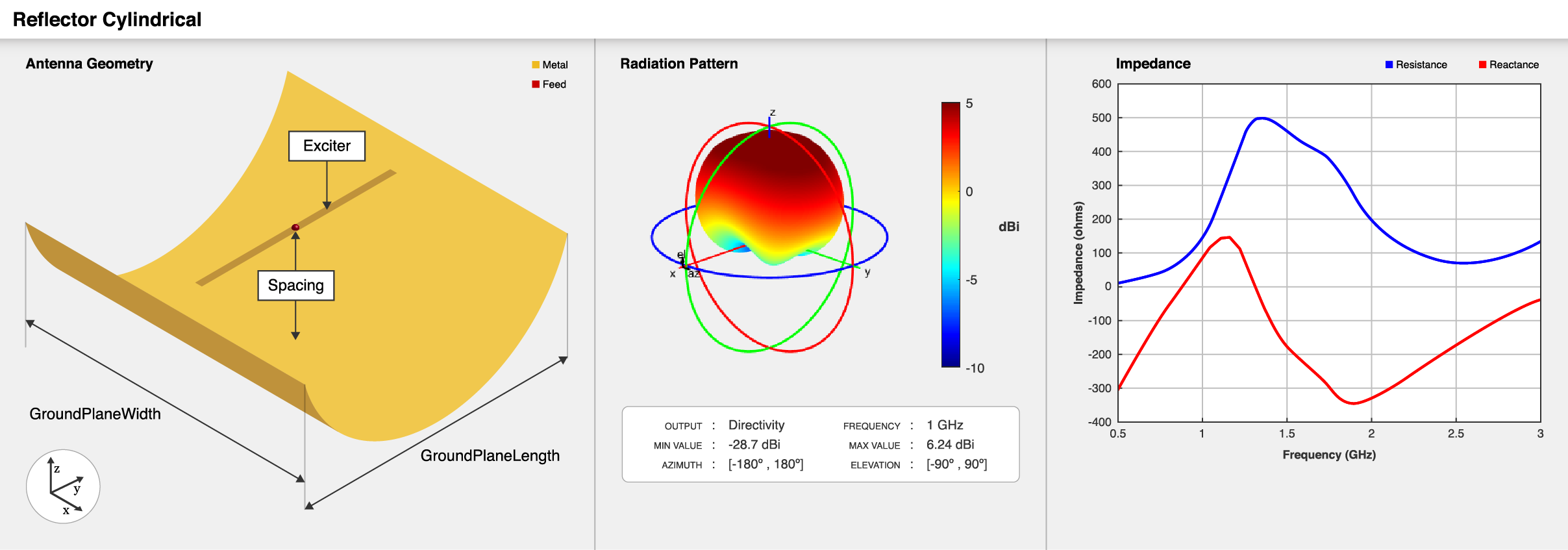## Creation

### Syntax

``ant = reflectorCylindrical``
``ant = reflectorCylindrical(Name,Value)``

### Description

example

````ant = reflectorCylindrical` creates a cylindrical reflector-backed antenna. The default cylindrical reflector antenna object has an exciter as a center-fed dipole located on the X-Y plane and the dimensions are chosen for an operating frequency of around 1 GHz.```

example

````ant = reflectorCylindrical(Name,Value)` sets Properties using name-value pairs. For example, `reflectorCylindrical('GroundPlaneWidth',0.21)` creates a cylindrical reflector with a width of 0.21 meters. You can specify multiple name-value pairs. Enclose each property name in quotes. Properties not specified retain their default values.```

## Properties

expand all

Antenna used as an exciter, specified as an `antenna` or an array object.

Example: `'Exciter',dipole`

Example: ```ant.Exciter = dipole('Length',0.1409,'Width',0.02,'FeedOffset',0,'Tilt',90,'TiltAxis',[0 1 0])```

Example: ```ant.Exciter = linearArray('patchMicrostrip')```

Reflector length along X-axis, specified as a positive scalar in meters.

Example: `'GroundPlaneLength',0.6`

Example: `ant.GroundPlaneLength = 0.18`

Data Types: `double`

Reflector width along Y-axis, specified as a positive scalar in meters.

Example: `'GroundPlaneWidth',0.4`

Example: `ant.GroundPlaneWidth = 0.18`

Data Types: `double`

Distance between reflector and exciter, specified as a scalar in meters.

Example: `'Spacing',0.059`

Example: `ant.Spacing = 0.195`

Data Types: `double`

Perpendicular distance between the ground plane and the aperture of the cylindrical reflector, specified as a positive scalar in meters.

Example: `'Depth',0.09`

Example: `ant.Depth = 0.049`

Note

`Depth` should be less than or equal to half of the GroundPlaneLength.

Data Types: `double`

Flag to enable the probe feed, specified as `0` or `1` . Setting the flag to `0` disables the probe feed, and setting the flag to `1` enables it.

Example: `'EnableProbeFeed',1`

Example: `ant.EnableProbeFeed = 1`

Data Types: `double`

Type of the metal used as a conductor, specified as a metal material object. You can choose any metal from the `MetalCatalog` or specify a metal of your choice. For more information, see `metal`. For more information on metal conductor meshing, see Meshing.

Example: `m = metal('Copper'); 'Conductor',m`

Example: `m = metal('Copper'); ant.Conductor = m`

Tilt angle of the antenna, specified as a scalar or vector with each element unit in degrees. For more information, see Rotate Antennas and Arrays.

Example: `Tilt=90`

Example: `Tilt=[90 90]`,`TiltAxis=[0 1 0;0 1 1]` tilts the antenna at 90 degrees about the two axes defined by the vectors.

Note

The `wireStack` antenna object only accepts the dot method to change its properties.

Data Types: `double`

Tilt axis of the antenna, specified as:

• Three-element vector of Cartesian coordinates in meters. In this case, each coordinate in the vector starts at the origin and lies along the specified points on the X-, Y-, and Z-axes.

• Two points in space, each specified as three-element vectors of Cartesian coordinates. In this case, the antenna rotates around the line joining the two points in space.

• A string input describing simple rotations around one of the principal axes, 'X', 'Y', or 'Z'.

Example: `TiltAxis=[0 1 0]`

Example: `TiltAxis=[0 0 0;0 1 0]`

Example: `TiltAxis = 'Z'`

Note

The `wireStack` antenna object only accepts the dot method to change its properties.

Data Types: `double`

Lumped elements added to the antenna feed, specified as a `lumpedElement` object. You can add a load anywhere on the surface of the antenna. By default, the load is at the feed. For more information, see `lumpedElement`.

Example: `'Load',lumpedelements`, where `lumpedelements` is the load added to the antenna feed.

Example: `ant.Load = lumpedElement('Impedance',75)`

## Object Functions

 `show` Display antenna, array structures or shapes `impedance` Input impedance of antenna; scan impedance of array `sparameters` Calculate S-parameter for antenna and antenna array objects `returnLoss` Return loss of antenna; scan return loss of array `vswr` Voltage standing wave ratio of antenna `optimize` Optimize antenna or array using SADEA optimizer `pattern` Radiation pattern and phase of antenna or array; Embedded pattern of antenna element in array `patternAzimuth` Azimuth pattern of antenna or array `patternElevation` Elevation pattern of antenna or array `axialRatio` Axial ratio of antenna `beamwidth` Beamwidth of antenna `current` Current distribution on antenna or array surface `charge` Charge distribution on antenna or array surface `efficiency` Radiation efficiency of antenna `EHfields` Electric and magnetic fields of antennas; Embedded electric and magnetic fields of antenna element in arrays `mesh` Mesh properties of metal, dielectric antenna, or array structure `design` Design prototype antenna or arrays for resonance around specified frequency `rcs` Calculate and plot radar cross section (RCS) of platform, antenna, or array

## Examples

collapse all

Create a cylindrical reflector antenna object with default properties.

`ant = reflectorCylindrical;`

View the antenna object.

`show(ant)`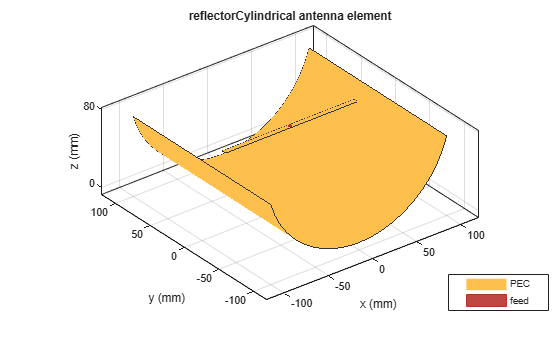Create a `reflectorCylindrical` antenna object with a rounded bow-tie dipole antenna as an exciter.

```b = bowtieRounded('Length',96e-3,'Tilt',90,'TiltAxis',[0 1 0]); r = reflectorCylindrical('Exciter',b,'Spacing',100e-3); ```

View the antenna object.

```figure show(r)```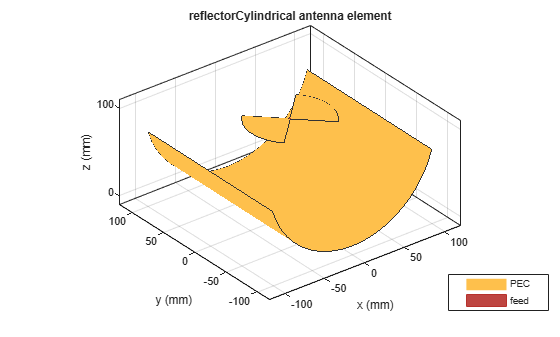Plot the radiation pattern at 1.5 GHz.

```figure pattern(r,1.5e9)```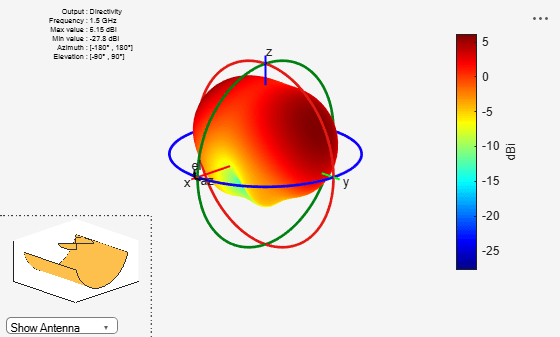Enable the probe feed for the `reflectorCylindrical` antenna object.

`re = reflectorCylindrical('Exciter',b,'Spacing',100e-3,'EnableProbeFeed',1);`

View the antenna object with the probe feed enabled.

```figure show(re)```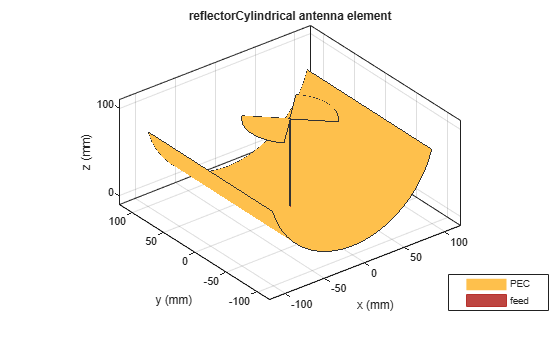Plot the radiation pattern of the antenna object at 1.5 GHz with the probe feed enabled.

```figure pattern(re,1.5e9)```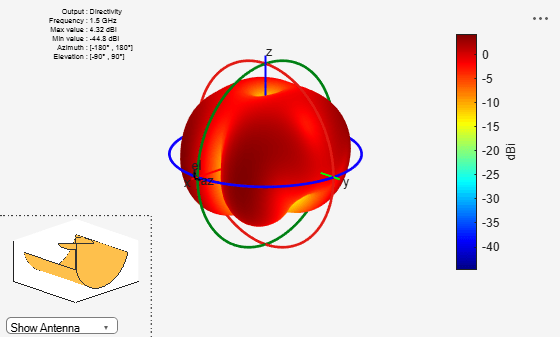Create a linear array of crossed dipole antenna.

```d = dipoleCrossed('Tilt',90,'TiltAxis',[0 1 0]); la = linearArray('Element',d,'NumElements',4,'ElementSpacing',0.05,'Tilt',90,'TiltAxis',[0 0 1]);```

Create a cylindrical reflector backed array.

`ant = reflectorCylindrical('Exciter',la,'Tilt',90)`
```ant = reflectorCylindrical with properties: Exciter: [1x1 linearArray] GroundPlaneLength: 0.2000 GroundPlaneWidth: 0.2000 Spacing: 0.0750 Depth: 0.0750 EnableProbeFeed: 0 Conductor: [1x1 metal] Tilt: 90 TiltAxis: [1 0 0] Load: [1x1 lumpedElement] ```
`show(ant)`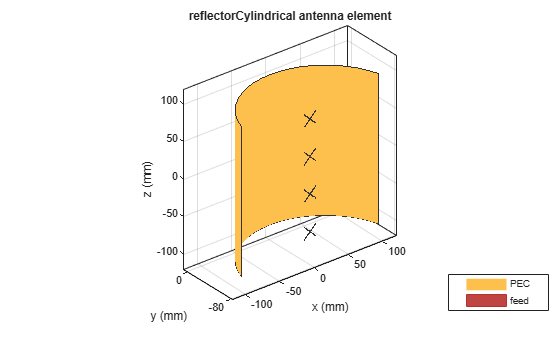Create a cylindrical reflector-backed Minkowski's loop fractal antenna.

```e = fractalIsland('Substrate',dielectric('Teflon'),'Tilt',90,'TiltAxis',[0 0 1]); ant = reflectorCylindrical('Exciter',e)```
```ant = reflectorCylindrical with properties: Exciter: [1x1 fractalIsland] GroundPlaneLength: 0.2000 GroundPlaneWidth: 0.2000 Spacing: 0.0750 Depth: 0.0750 EnableProbeFeed: 0 Conductor: [1x1 metal] Tilt: 0 TiltAxis: [1 0 0] Load: [1x1 lumpedElement] ```
`show(ant)`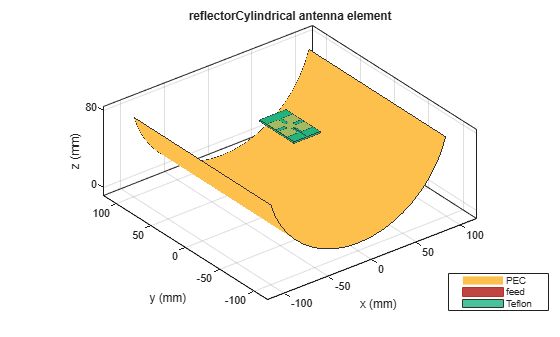Balanis, Constantine A. Antenna Theory: Analysis and Design. 3rd ed. Hoboken, NJ: John Wiley, 2005.